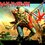# Pythogoreans vs Infinite descentimg

The regular pentagram was the badge of the Pythogoreans.But the Pythagoreans first thought that all the rations were rational,i.e,$x = \frac{a}{b}$. where a nd b are rational numbers.

Clearly this fraction must follow the equation for the golden ratio(It's a property of the pentagram),and hence we get $a^{2} = ab+b^{2}$

If $a$ and $b$ are of different parity clearly this leads to a contradiction.Also the assumption that $a$ and $b$ are odd also leads to a contradiction.

Hence both $a$ and $b$ must be even,that is $a = 2a_1$ and $b = 2b_1$,where $a_1 < a$ and $b_1 < b$.

Substituting this into the equation and cancelling we get $a_1^{2} = a_1b_1 + b_1^{2}$.

Now the same logic may be applied to this equation also which gives $a_1 = 2a_2$ and $b_1 = 2b_2$ where $a_2 and $b_2 < b_1$.

So we obtain an infinitely decreasing sequence of positive integers,ie $a>a_1>a_2>a_3>.....$ and $b>b_1>b_2>b_3>...........$.

Clearly no such infinitely decreasing sequence exists for positive integers.

For more details on this approach you may choose to read my note on Fermat's Method of Infinite Descent7 years, 1 month ago

This discussion board is a place to discuss our Daily Challenges and the math and science related to those challenges. Explanations are more than just a solution — they should explain the steps and thinking strategies that you used to obtain the solution. Comments should further the discussion of math and science.

When posting on Brilliant:

• Use the emojis to react to an explanation, whether you're congratulating a job well done , or just really confused .
• Ask specific questions about the challenge or the steps in somebody's explanation. Well-posed questions can add a lot to the discussion, but posting "I don't understand!" doesn't help anyone.
• Try to contribute something new to the discussion, whether it is an extension, generalization or other idea related to the challenge.

MarkdownAppears as
*italics* or _italics_ italics
**bold** or __bold__ bold
- bulleted- list
• bulleted
• list
1. numbered2. list
1. numbered
2. list
Note: you must add a full line of space before and after lists for them to show up correctly
paragraph 1paragraph 2

paragraph 1

paragraph 2

[example link](https://brilliant.org)example link
> This is a quote
This is a quote
    # I indented these lines
# 4 spaces, and now they show
# up as a code block.

print "hello world"
# I indented these lines
# 4 spaces, and now they show
# up as a code block.

print "hello world"
MathAppears as
Remember to wrap math in $$ ... $$ or $ ... $ to ensure proper formatting.
2 \times 3 $2 \times 3$
2^{34} $2^{34}$
a_{i-1} $a_{i-1}$
\frac{2}{3} $\frac{2}{3}$
\sqrt{2} $\sqrt{2}$
\sum_{i=1}^3 $\sum_{i=1}^3$
\sin \theta $\sin \theta$
\boxed{123} $\boxed{123}$

Sort by:

Nice note! But in my opinion, the note jumps out on you, does its job and then runs away.

Additional background, like what the golden ratio is, what a pentagram is and how these two are related would have been helpful.

This note proves that golden ratio can not be expressed in the form $\frac{a}{b}$ where $a$ and $b$ are positive integers. But it does not explicitly mentions this statement anywhere.

Despite all this, this is a nice demonstration of proofs that use the method infinite descent.

- 7 years, 1 month ago

Thanks for the constructive review.Actually I didn't include the golden ration in this note because many people have posted about it before. Here is my note on Stochastic Programming.It will be extremely helpful for me if you write a small review of that note too.....I put a lot of effort into this one but it didn't get attention.Thanks in advance.

- 7 years, 1 month ago

can we say that infinite descent is a strong form of pentagram?

- 7 years, 1 month ago

The symbol is the pentagram and infinite descent is the proof technique....clck on the link if you're interested to learn more about it...

- 7 years, 1 month ago